Home MonkeyNotes Printable Notes Digital Library Study Guides Study Smart Parents Tips College Planning Test Prep Fun Zone Help / FAQ How to Cite New Title Request

 Distance Formula 1) Distance of a point p (x1, y1) from the origin O, is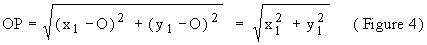2) Distance between two points A(x1,y1) and B(x2,y2) , is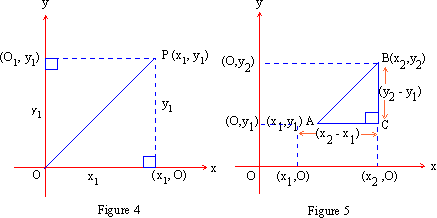Mid point formula The midpoint of the segment between (x1,y1) and (x2, y2) is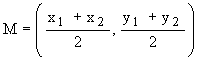Which is clear from the Figure 6 and Figure 7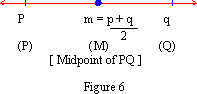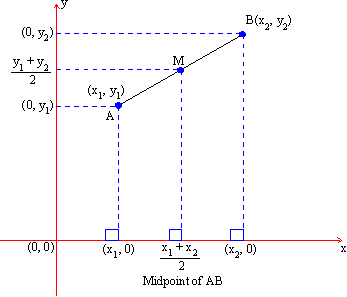Figure 7 Example Find the midpoint of the segment between (-8, 2) and (6, 4) Solution : Let A(x1, y1) = (-8, 2) and C (x2, y2) = (6,4) Let B (x, y) be the midpoint of the Segment AC then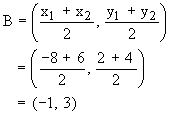Example Find the length of the median through A, for the triangle A(7, -3), B(3, -1) and C(5, 3). Solution : Let D be the midpoint of BC. Then the co-ordinates of D are :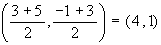Hence, median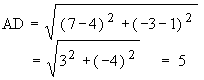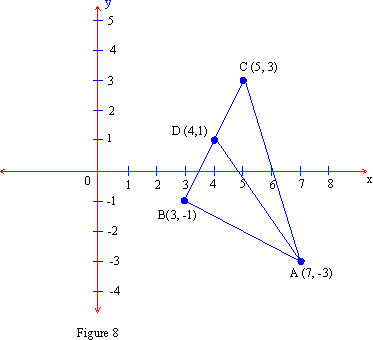Index 8.1 Definition8.2 Relations, Graphs and Symmetry8.3 Slopes and Intercepts Chapter 9
 Search: All Products Books Popular Music Classical Music Video DVD Toys & Games Electronics Software Tools & Hardware Outdoor Living Kitchen & Housewares Camera & Photo Cell Phones Keywords: